# Van der Pol equation

The non-linear second-order ordinary differential equation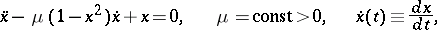(1)

which is an important special case of the Liénard equation. Van der Pol's equation describes the auto-oscillations (cf. Auto-oscillation) of one of the simplest oscillating systems (the van der Pol oscillator). In particular, equation (1) serves — after making several simplifying assumptions — as a mathematical model of a generator on a triode for a tube with a cubic characteristic. The character of the solutions of equation (1) was first studied in detail by B. van der Pol .

Equation (1) is equivalent to the following system of two equations in two phase variables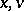: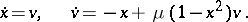(2)

It is sometimes convenient to replace the variableby the variable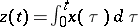; equation (1) then becomes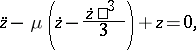which is a special case of the Rayleigh equation. If, together with, one also considers the variable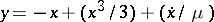, introduces a new time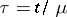and puts, one obtains the system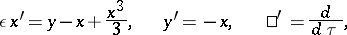(3)

instead of equation (1). For any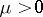there exists a unique stable limit cycle in the phase plane of the system (2) to which all other trajectories (except for the equilibrium position at the coordinate origin) converge as; this limit cycle describes the oscillations of the van der Pol oscillator , , .

For smallthe auto-oscillations of the oscillator (1) are close to simple harmonic oscillations (cf. Non-linear oscillations) with period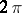and specified amplitude. In order to calculate the oscillation process more accurately, asymptotic methods are employed. Asincreases, the auto-oscillations of the oscillator (1) deviate more and more from harmonic oscillations. Ifis large, equation (1) describes relaxation oscillation with period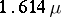(to a first approximation). More accurate asymptotic expansions of magnitudes characterizing relaxation oscillations  are known: The study of these oscillations is equivalent to the study of the solutions of the system (3) with a small coefficientin front of the derivative .

The equation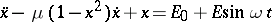describes the behaviour of the van der Pol oscillator when acted upon by a periodic external disturbance. The most important in this context is the study of frequency capture (the existence of periodic oscillations), beats (the possibility of almost-periodic oscillations) and chaotic behaviour , .